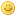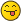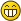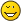Pentation roots self but please you do...nuninho1980FellowPosts: 98 Threads: 6 Joined: Apr 200910/18/2010, 12:09 AM (This post was last modified: 10/18/2010, 12:12 AM by nuninho1980.) $y = \text{Pentaroot}_x (b) = b /\uparrow\uparrow\uparrow x \Leftrightarrow b = y \uparrow\uparrow\uparrow x$ $\text{Pentaroot}_2 (2) = ?$ $\text{Pentaroot}_3 (3) = ?$ $\text{Pentaroot}_4 (4) = ?$ $\text{Pentaroot}_5 (5) = ?$ $\lim_{x\to\infty}\text{Pentaroot}_x (x) = ?$ By the results have 5 cases decimais (minimum). Please you think to calculate on up... I have known its by program Pari/GP (it's very fast).x)sheldonisonLong Time FellowPosts: 684 Threads: 24 Joined: Oct 2008 11/03/2010, 05:26 AM (10/18/2010, 12:09 AM)nuninho1980 Wrote: $y = \text{Pentaroot}_x (b) = b /\uparrow\uparrow\uparrow x \Leftrightarrow b = y \uparrow\uparrow\uparrow x$ $\text{Pentaroot}_2 (2) = ?$ $\text{Pentaroot}_3 (3) = ?$ $\text{Pentaroot}_4 (4) = ?$ $\text{Pentaroot}_5 (5) = ?$ $\lim_{x\to\infty}\text{Pentaroot}_x (x) = ?$ By the results have 5 cases decimais (minimum). Please you think to calculate on up... I have known its by program Pari/GP (it's very fast).x)Pentation is hard to understand.... Here's my results. I used "b" for the base. $b\uparrow\uparrow\uparrow n = n$, for each "n", calculate b $\text{sexp}_b(\text{sexp}_b(1))=2$, calculate b $\text{sexp}_b(\text{sexp}_b(\text{sexp}_b(1)))=3$, calculate b $\text{sexp}_b(\text{sexp}_b(\text{sexp}_b(\text{sexp}_b(1))))=4$, calculate b and so on, limit as $n \to \infty$ n= 2 1.63221539635499 n= 3 1.73480823757765 n= 4 1.73013167405422 n= 5 1.71198477313212 n= 6 1.69588829898111 n=70 1.63599652477221 I calculated these values by simple binary search, but I used "\p 28", which is accurate to ~14 digits, but very fast, 4 seconds for init(B);loop. Only problem is its very easy to get an overflow, so the initial starting based needs to readjusted; for n=70, I used a more complicated algorithm. Code:\r kneser.gp \p 28; { curbase=1.6;   curstep=0.1;   while ((curstep>1E-14),     init(curbase);loop;     y = sexp(sexp(B));     if (y>3, curbase=curbase-curstep, curbase=curbase+curstep);     curstep=curstep/2;   ); } As n goes to infinity, I would expect the value for b to go to Nuinho's constant, the base for which the upper fixed point of sexp is parabolic, b=1.635324496715276399345344618306171 - Sheldonnuninho1980FellowPosts: 98 Threads: 6 Joined: Apr 2009 11/03/2010, 12:54 PM (This post was last modified: 11/03/2010, 12:56 PM by nuninho1980.) It's excellent!! Congratulations!But you are 2 weeks later. I already could calculate pentarootsbut I tried to "explore" each digit by "lottery" up to 5 digits from days 15 to 17, october because I used only "kneser.gp".Nuinho's constant - bad but yes Nuninhothank!« Next Oldest | Next Newest »

 Possibly Related Threads… Thread Author Replies Views Last Post On extension to "other" iteration roots Leo.W 34 9,678 08/30/2022, 03:29 AM Last Post: JmsNxn Pentation fractal Daniel 11 2,880 08/03/2022, 03:04 AM Last Post: JmsNxn pentation and hexation sheldonison 9 24,666 09/18/2019, 02:34 PM Last Post: sheldonison Solving tetration using differintegrals and super-roots JmsNxn 0 4,370 08/22/2016, 10:07 PM Last Post: JmsNxn Tetration is pentation. This deserve more thinking. marraco 2 8,216 03/30/2015, 02:54 PM Last Post: marraco Mizugadro, pentation, Book Kouznetsov 41 109,061 03/02/2015, 08:13 PM Last Post: sheldonison Infinite Pentation (and x-srt-x) andydude 20 51,936 05/31/2011, 10:29 PM Last Post: bo198214 Regular "pentation"? mike3 12 38,356 04/04/2011, 03:16 AM Last Post: BenStandeven proof: Limit of self-super-roots is e^1/e. TPID 6 bo198214 3 12,926 07/10/2010, 09:13 AM Last Post: bo198214 Pentation's definitional ambiguity Base-Acid Tetration 14 41,471 12/15/2009, 11:23 PM Last Post: Base-Acid Tetration

Users browsing this thread: 1 Guest(s)